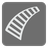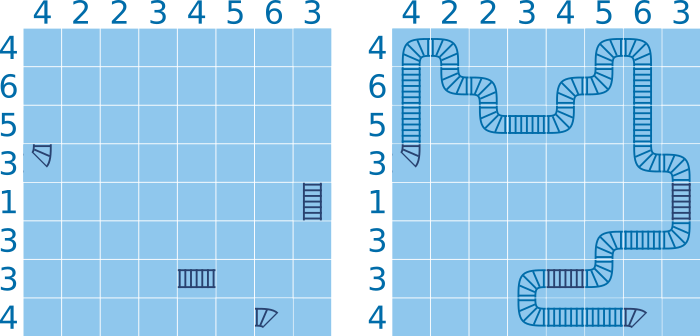Train Tracks
Undefined
English

This mission is an adaptation of the "Tracks" game (from Simon Tatham's Portable Puzzle Collection ). If you are lost or just want to play, the game is available here .

You have to trace train tracks from "start" to "end" in a rectangular grid, respecting constraints:

• Each row and column must contain a given number of tracks.
• Some tracks are fixed and you must use them as they are. Their positions are given with the entry / exit directions (North / South / West / East). You can choose the entry.
• The exit direction of the start, and the entry direction of the end are fixed.
• The track may not cross itself.
You will have to return how you move from start to end with North / South / West / East directions.

• Rows (and columns) count constraints will be represented by a list of integers.
• Start (and end) will be represented by its coordinates, a tuple of two integers.
• Fixed tracks will be represented by a dictionary with coordinates (a tuple of two integers) as keys and a set of entry / exit directions as values.
Note: ``` (len(rows), len(columns)) ``` give the grid dimensions.

Input: Two lists of integers, two tuples of two integers and a dictionary (keys: a tuple of two integers; values: a set of one or two strings).

Output: A string.

Example:

```Your moves from (3, 0) to (7, 6): "NNNESESEENENESSSESSWWSWWSEEE".
```
```train_tracks([4, 6, 5, 3, 1, 3, 3, 4],  # rows
[4, 2, 2, 3, 4, 5, 6, 3],  # columns
(3, 0),                    # start
(7, 6),                    # end
{(3, 0): {'N'},            # constraints
(4, 7): {'N', 'S'},
(6, 4): {'E', 'W'},
(7, 6): {'W'}},
) == 'NNNESESEENENESSSESSWWSWWSEEE'
```

To play the puzzles / tests yourself: 1 2 3 4 5 6 7 8 9 10 11 12 13 14 15 16 17

Preconditions:

• All puzzles are solvable.
• 8 ≤ len(rows) ≤ 50 and 8 ≤ len(columns) ≤ 50.
• all(0 ≤ row < len(rows) and 0 ≤ col < len(columns) for row, col in constraints)
• all(dirs ≤ {'E', 'N', 'S', 'W'} and 1 ≤ len(dirs) ≤ 2 for dirs in constraints.values())
• {coords for coords, dirs in constraints.items() if len(dirs) == 1} == {start, end}